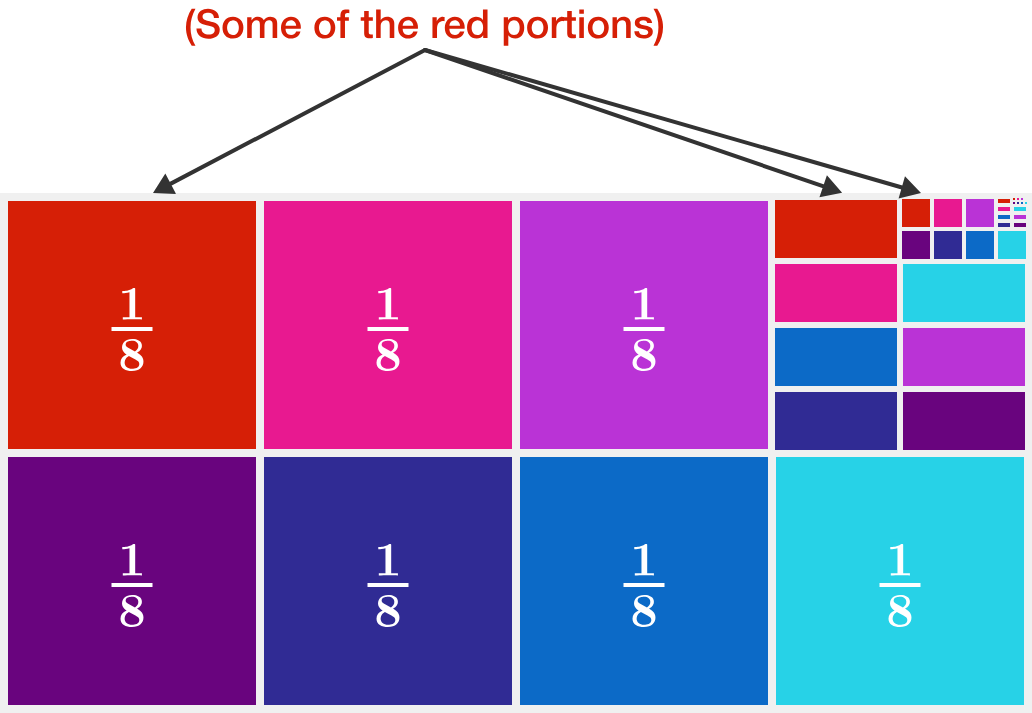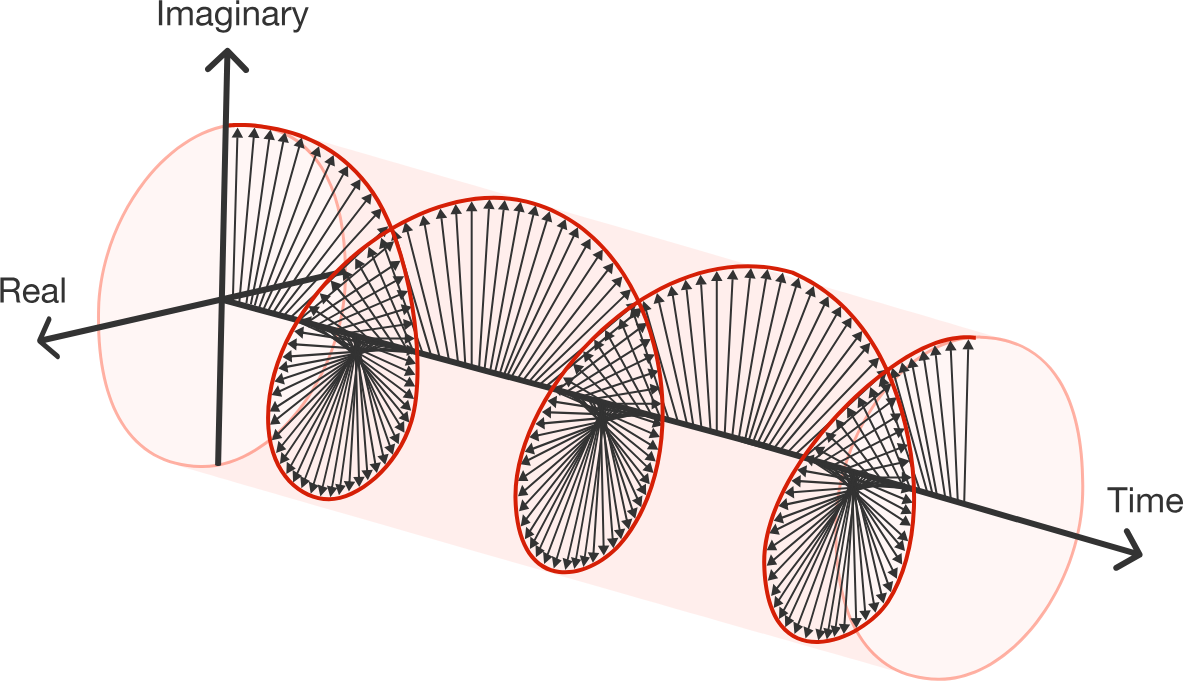### Complex Numbers

Before we dive into Euler's formula and Complex Algebra, let's test your intuitive understanding of non-complex algebra.

\begin{aligned} \large 9^a&\large= 25 \\ \\ \large 3^a &\large = \ ? \end{aligned}

What goes at the question mark in the equations above?

Hint: There's a much faster way than solving for $a.$ You are not a robot, and you don't need a calculator to solve this!# Algebraic Intuition

$\large \frac { 1 }{ { 2 }^{ 3 } } +\frac { 1 }{ { 2 }^{ 6 } } +\frac { 1 }{ { 2 }^{ 9 } } + \cdots = \ ?$

Hint: Don’t try this one on a calculator either - your fingers will wear out! This algebra problem can be solved with a geometric shortcut:What total fraction of this image is red? (Each "upper left square" portion in each iteration is included.)

# Algebraic Intuition

Which is greater, $A$ or $B?$

$A=\frac{99^{999}+1}{99^{1000}+1}$

$B=\frac{99^{1000}+1}{99^{1001}+1}$

# Algebraic Intuition

$\log_2 3$ $\times \log_3 4$ $\times \log_4 5$ $\times \log_5 6$ $\times \log_6 7$ $\times \log_7 8 = \, ?$# Algebraic Intuition

If $x$ is a real number satisfying the equation $\sqrt {x + 15} + \sqrt x = 15,$ find the value of $x$.

# Algebraic Intuition

Nice work!

Next we'll dive into some complex algebra and the motivations for studying it. But, in all of these cases, your intuitive understanding of algebra will be the basis for solving problems. Even complex algebra is first and foremost algebra. Whether you're working with complex numbers, exponents, trigonometric functions, etc. we'll be exploring how to skillfully manipulate these mathematical concepts in algebraic ways.×